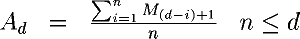Next: Weighted moving averages Up: Calculating moving averages Previous: Calculating moving averages

### Simple moving averages

The n day simple moving average for day d is computed by:If we have ten measurements, M1 through M10, and we wish to compute a four day moving average, the moving averages for successive days are:We can't compute a four day moving average until we have four days worth of data. That's why the first moving average in this example is A4.

By John Walker